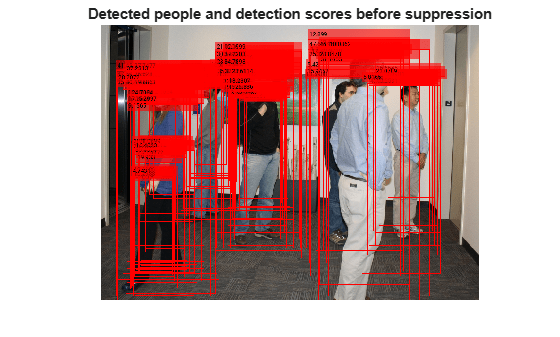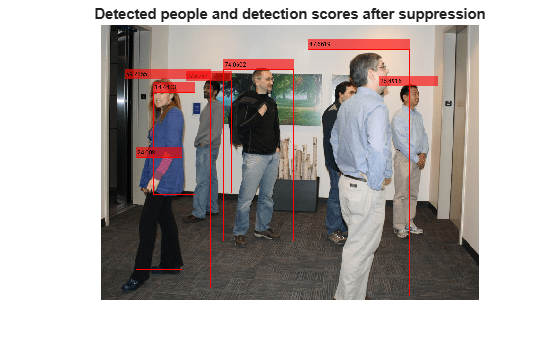# selectStrongestBbox

Select strongest bounding boxes from overlapping clusters using nonmaximal suppression (NMS)

## Syntax

``````[selectedBbox,selectedScore] = selectStrongestBbox(bbox,score)``````
``````[selectedBbox,selectedScore,index] = selectStrongestBbox(bbox,score)``````
``````[___] = selectStrongestBbox(___,Name,Value)``````

## Description

example

``````[selectedBbox,selectedScore] = selectStrongestBbox(bbox,score)``` returns selected bounding boxes that have a high confidence score. The function uses nonmaximal suppression (NMS) to eliminate overlapping bounding boxes from the `bbox` input.```
``````[selectedBbox,selectedScore,index] = selectStrongestBbox(bbox,score)``` additionally returns the `index` vector associated with `selectedBbox`. This vector contains the indices of the selected boxes in the `bbox` input.```
``````[___] = selectStrongestBbox(___,Name,Value)``` uses additional options specified by one or more `Name,Value` pair arguments.```

## Examples

collapse all

Load the pretrained aggregate channel features (ACF) people detector.

`peopleDetector = peopleDetectorACF();`

Detect people in an image. Disable the default nonmaximal suppression used by the detector.

```I = imread('visionteam1.jpg'); [bbox,score] = detect(peopleDetector,I,'SelectStrongest',false); ```

Run nonmaximal suppression with custom threshold.

`[selectedBbox,selectedScore] = selectStrongestBbox(bbox,score,'OverlapThreshold',0.3);`

Display the results.

```I1 = insertObjectAnnotation(I,'rectangle',bbox,score,'AnnotationColor','r'); I2 = insertObjectAnnotation(I,'rectangle',selectedBbox,selectedScore,'AnnotationColor','r'); figure, imshow(I1); title('Detected people and detection scores before suppression'); ``````figure, imshow(I2); title('Detected people and detection scores after suppression');```## Input Arguments

collapse all

Bounding boxes, specified as an M-by-4 or M-by-5 nonsparse numeric matrix. M is the number of bounding boxes. Each row of the matrix defines a bounding box as either an axis-aligned rectangle or a rotated rectangle. This table describes the format for each bounding box.

Bounding BoxDescription
Axis-aligned rectangle

Defined in spatial coordinates as an M-by-4 numeric matrix with rows of the form [x y w h], where:

• M is the number of axis-aligned rectangles.

• x and `y` specify the upper-left corner of the rectangle.

• w specifies the width of the rectangle, which is its length along the x-axis.

• h specifies the height of the rectangle, which is its length along the y-axis.

Rotated rectangle

Defined in spatial coordinates as an M-by-5 numeric matrix with rows of the form [xctr yctr xlen ylen yaw], where:

• M is the number of rotated rectangles.

• xctr and yctr specify the center of the rectangle.

• xlen specifies the width of the rectangle, which is its length along the x-axis before rotation.

• ylen specifies the height of the rectangle, which is its length along the y-axis before rotation.

• yaw specifies the rotation angle in degrees. The rotation is clockwise-positive around the center of the bounding box.Data Types: `single` | `double` | `int8` | `int16` | `int32` | `uint8` | `uint16` | `uint32`

Confidence score, specified as an M-by-1 vector. The Mth score corresponds to the Mth bounding box in the `bbox` input. The `selectStrongestBbox` function uses nonmaximal suppression to eliminate overlapping bounding boxes and associate the confidence score with the boxes. A higher score represents a higher confidence in keeping the bounding box. The `score` input must be real, finite, and nonsparse.

Data Types: `single` | `double` | `int8` | `int16` | `int32` | `uint8` | `uint16` | `uint32`

### Name-Value Arguments

Specify optional pairs of arguments as `Name1=Value1,...,NameN=ValueN`, where `Name` is the argument name and `Value` is the corresponding value. Name-value arguments must appear after other arguments, but the order of the pairs does not matter.

Before R2021a, use commas to separate each name and value, and enclose `Name` in quotes.

Example: `'RatioType'`,`'Union'` sets the `'RatioType'` property to `'Union'`.

Ratio type, specified as the character vector `'Union'` or `'Min'`.

• Set the ratio type to `'Union'` to compute the ratio as the area of intersection between `bboxA` and `bboxB`, divided by the area of the union of the two.

• Set the ratio type to `'Min'` to compute the ratio as the area of intersection between `bboxA` and `bboxB`, divided by the minimum area of the two bounding boxes.Data Types: `char`

Overlap ratio threshold, specified as the comma-separated pair consisting of '`OverlapThreshold`' and a scalar in the range [0 1]. When the overlap ratio is above the threshold you set, the function removes bounding boxes around the reference box. Decrease this value to reduce the number of selected bounding boxes. However, if you decrease the overlap ratio too much, you might eliminate boxes that represent objects close to each other in the image.

Data Types: `single` | `double`

Maximum number of strongest boxes, specified as the comma-separated pair consisting of `'NumStrongest'` and `inf` or a positive scalar. Use this argument to reduce processing time when you have a priori knowledge about the maximum number of boxes. Set the value to `inf` to select all the strongest, non-overlapping, bounding boxes.

## Output Arguments

collapse all

Selected bounding boxes, returned as an M-by-4 or an M-by-5 matrix. The 4-element vectors represent axis-aligned rectangles and the 5-element vectors represent rotated rectangles.

The `selectedBbox` output returns the selected bounding boxes from the `bbox` input that have the highest confidence score. The function uses nonmaximal suppression to eliminate overlapping bounding boxes.

Data Types: `single` | `double` | `int8` | `int16` | `int32` | `uint8` | `uint16` | `uint32`

Scores of selected bounding boxes, returned as an M-by-1 vector. The Mth score in the `selectedScore` output corresponds to the Mth bounding box in the `selectedBbox` output.

Data Types: `single` | `double` | `int8` | `int16` | `int32` | `uint8` | `uint16` | `uint32`

Index of selected bounding boxes, returned as an M-by-1 vector. The `index` vector contains the indices to the selected boxes in the `bbox` input.

Data Types: `double`

## Version History

Introduced in R2014b